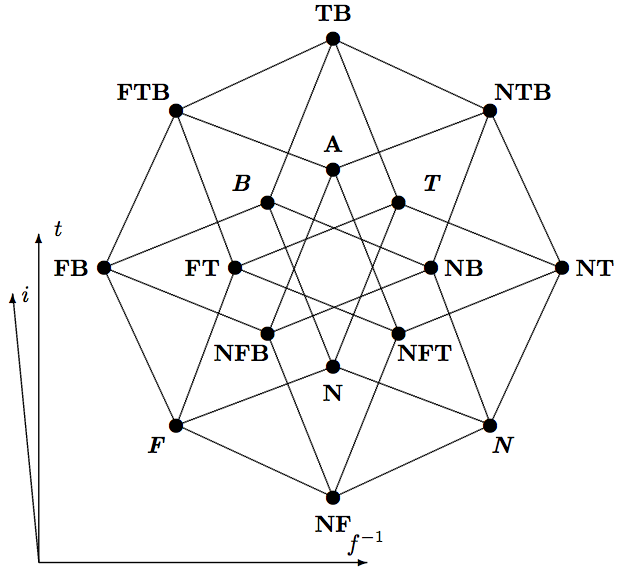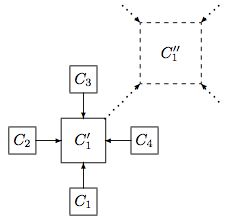## Generalized Truth Values and Multilattices

It is possible to generalize the notion of a bilattice by introducing the notion of a multilattice, which is suitable for investigating sets of generalized truth values in the presence of many partial orderings defined on these sets.

Definition. An n-dimensional multilattice (or simply n-lattice) is a structure $$\mathcal{M}_n = \langle S, \le_1 ,\ldots ,\le_n\rangle$$, where $$S$$ is a non-empty set and $$\le_1 ,\ldots ,\le_n$$ are partial orders defined on $$S$$ such that $$(S, \le_1),\ldots ,(S, \le_n)$$ are all distinct lattices.

In particular, if one applies the idea of a generalized truth value function to Belnap’s four truth values, then one obtains valuations assigning the 16 generalized truth values from the powerset $$\mathcal{P}(\mathbf{4}) = \mathbf{16}$$ of $$\mathbf{4}$$:

\begin{align} \text{1.}&& \mathbf{N} &= \varnothing& \text{9.}&& \mathbf{FT} &= \{\{F\}, \{T\}\}\\ \text{2.}&& \boldsymbol{N} &= \{\varnothing \}& \text{10.}&& \mathbf{FB} &= \{\{F\}, \{F, T\}\}\\ \text{3.}&& \boldsymbol{F} &= \{\{F\}\}& \text{11.}&& \mathbf{TB} &= \{\{T\}, \{F, T\}\}\\ \text{4.}&& \boldsymbol{T} &= \{\{T\}\}& \text{12.}&& \mathbf{NFT} &= \{\varnothing , \{F\}, \{T\}\}\\ \text{5.}&& \boldsymbol{B} &= \{\{F, T\}\}& \text{13.}&& \mathbf{NFB} &= \{\varnothing , \{F\}, \{F, T\}\}\\ \text{6.}&& \mathbf{NF} &= \{\varnothing , \{F\}\}& \text{14.}&& \mathbf{NTB} &= \{\varnothing , \{T\}, \{F, T\}\}\\ \text{7.}&& \mathbf{NT} &= \{\varnothing , \{T\}\}& \text{15.}&& \mathbf{FTB} &= \{\{F\}, \{T\}, \{F, T\}\}\\ \text{8.}&& \mathbf{NB} &= \{\varnothing , \{F, T\}\}& \text{16.}&& \mathbf{A} &= \{\varnothing , \{T\}, \{F\}, \{F, T\}\}.\\ \end{align}

These values give rise to an algebraic structure with three distinct partial orders: an information order $$\le_i$$ (viz. set-inclusion), a truth order $$\le_t$$ and a falsity order $$\le_f$$. Whereas the truth order is defined in terms of the presence and absence of the classical value $$T$$ in/from elements from $$\mathbf{16}$$, the falsity order is defined in terms of the presence and absence of $$F$$ in/from elements from $$\mathbf{16}$$ (see Shramko and Wansing 2005, 2006). The resulting algebraic structure is known as the trilattice SIXTEEN$$_3$$, which is presented by a triple Hasse diagram in Figure 4 (essentially the same structure has been introduced in Shramko, Dunn, Takenaka 2001 as a truth value space of constructive truth values).Figure 4: Trilattice SIXTEEN$$_3$$

This set of values can serve as a natural semantic foundation for the logic of a simple computer network. Indeed, one can observe that Belnap’s “computerized” interpretation works perfectly well only if we deal with one (isolated) computer receiving information from classical sources, i.e., these sources operate exclusively with the classical truth values. As soon as a computer $$C$$ is connected to other computers, there is no reason to assume that these computers cannot pass higher-level information concerning a given proposition to $$C$$. If several computers form a computer network, Belnap’s ideas that motivated $$\mathbf{B}_4$$ can be generalized. Consider, for example, four computers: $$C_1, C_2, C_3$$, and $$C_4$$ connected to another computer $$C_1 '$$, a server, to which they are supposed to supply information (Figure 5).Figure 5: A computer network

It turns out that the logic of the server itself (so, the network as a whole) cannot remain four-valued any more. Indeed, suppose $$C_1$$ informs $$C_1 '$$ that a sentence is true only (has the value $$\mathbf{T})$$, whereas $$C_2$$ supplies inconsistent information (the sentence is both true and false, i.e., has the value $$\mathbf{B})$$. In this situation $$C_1 '$$ has received the information that the sentence simultaneously is true only (i.e., true and not false) as well as both true and false, in other words, it has a value not from $$\mathbf{4}$$, but from $$\mathcal{P}(\mathbf{4})$$, namely the value $$\mathbf{TB} = \{\{T\}, \{T, B\}\}$$. Note, that this new value cannot simply be reduced to Belnap’s value $$\mathbf{B}$$, at least not without some “forced argument” and a serious information loss (see detailed explanations in Shramko and Wansing 2005: 124). Thus, if $$C_1 '$$ has been informed simultaneously by $$C_1$$ that a sentence is true-only, by $$C_2$$ that it is false-only, by $$C_3$$ that it is both-true-and-false, and by $$C_4$$ that it is neither-true-nor-false, then the value $$\mathbf{NFTB} = \{\varnothing , \{T\}, \{F\}, \{T, F\}\}$$ is far from being a “madness” (cf. Meyer 1978: 19) but is just an adequate value which should be ascribed to the sentence by $$C_1 '$$. That is, the logic of $$C_1 '$$ has to be 16-valued.

It is worth noticing that whereas in the bilattice FOUR$$_2$$ the logical order is not merely a truth order, but rather a truth-and-falsity order (an increase in truth means here a simultaneous decrease in falsity), the trilattice SIXTEEN$$_3$$ makes it possible to discriminate between a truth order and a (non-)falsity order, as it is shown in Figure 4. This means that in SIXTEEN$$_3$$, in addition to the information order (namely the subset relation), we have actually two distinct logical orders: one for truth, $$\le_t$$, and one for falsity, $$\le_f$$. Both of these logical orderings determine their own algebraic operations of meet, joint and inversion, and thus two distinct, although strictly “parallel”, sets of logical connectives (for conjunctions, disjunctions and negations). Moreover, both of these orderings also determine their own logic, one in a truth vocabulary (where entailment and logical connectives are defined with respect to $$\le_t)$$, and another in a falsity vocabulary (where entailment and connectives are defined with respect to $$\le_f)$$. It turns out that for both languages one obtains first-degree entailment as the logic of SIXTEEN$$_3$$ (see Shramko and Wansing 2005). In Shramko and Wansing 2006 this observation has been generalized to trilattices of any degree. That is, if the above network is extended so that the computer $$C_1 '$$ may pass information to another computer $$(C_1 '')$$, then the amount of semantical values will increase to $$2^{16} = 65536$$, and so on. Nevertheless, this exponential growth of the number of truth values turns out to be unproblematic, because the logic of the generalized so-called Belnap trilattices in the truth vocabulary as well as in the falsity vocabulary always is first-degree entailment, see also Beall and Camrud 2020).

Concerning the full language that combines both the truth vocabulary (with conjunction, disjunction and negation defined with respect to $$\le_t)$$ and the falsity vocabulary (with conjunction, disjunction and negation defined with respect to $$\le_f)$$, the problem of axiomatizing the truth and falsity consequence relations determined by the truth and falsity orderings on the trilattice SIXTEEN$$_3$$ remained open for a couple of years. A first solution was found by Odintsov (2009), for extended languages that contain a truth (falsity) implication defined as the so-called residuum of the truth (falsity) ordering on SIXTEEN$$_3$$. The presence of such an implication connective allows one to reduce the truth (falsity) entailment relation to the set of tautologies, which are defined as formulas that under any interpretation are evaluated as the greatest element with respect to the truth (falsity) ordering. The axiomatization problem has finally been solved in (Odintsov and Wansing 2015) by showing that the logic of SIXTEEN$$_3$$ is the logic of commutative distributive bilattices. This result was further extended to any multilattices in (Shramko 2016).

Thomas Ferguson (2017) has shown that the logic of bilattices equipped with the so-called “cut-down operations” (operations that “cut-down” the field of available data) introduced by Melvin Fitting is equivalent to Harry Deutsch’s (1984) four-valued system now called S$$_{fde}$$ (see also Oller 1999), which is a first-degree entailment fragment of Parry’s analytic implication. Thus, exactly as E$$_{fde}$$ serves as the logic of all logical bilattices, S$$_{fde}$$ plays an analogous role for bilattices with cut-down operations. This result can be generalized with respect to trilattices and further to multilattices with cut-down operations.

Recently Omori and Skurt (2019) have offered two alternative semantical presentations for SIXTEEN$$_3$$ based on certain combinations of Routley’s and Dunn’s semantical constructions.